# Excel TODAY function to insert today's date and more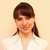by , updated on

The tutorial shows how to insert today's date in your worksheets and demonstrates a few other uses of TODAY function in Excel.

You want to put today's date in some cell? Or you wish to highlight the current date in your Excel calendar? Or you'd like to find the date closest to today? All this can be done by using the Excel TODAY function and this tutorial will teach you how.

## Excel TODAY function - syntax and basic uses

The TODAY function in Excel does exactly what its name indicates - returns today's date.

The syntax of the TODAY function is as simple as it could possible be - it does not have any arguments at all. Whenever you need to insert today's date in Excel, just enter the following formula in a cell:

`=TODAY()`

You can format the value returned by TODAY in any built-in or custom date format. For example, this way: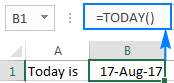### 4 things you should know about TODAY in Excel

1. TODAY() is a volatile function, which means that it updates itself continuously every time a worksheet is opened or changed.
2. If a TODAY formula does not update automatically, most likely automatic recalculation is turned off in your workbook. To turn it on again, go to the Formulas tab > Calculation Options, and select Automatic.
3. To enter today's date in Excel as a static unchangeable value, use these keyboard shortcuts.
4. If you want to insert current date and time, use the NOW function instead of TODAY.

## How to insert today's date in Excel

There are two ways to enter the current date in Excel - a formula and shortcut. Which one to use depends on whether you want a static or dynamic value.

### Excel formula for today's date

The value returned by the TODAY function updates automatically, so the below formula is useful if you want the worksheet to always display the current date, regardless of when you open it.

`=TODAY()`

To clarify what kind of date that is, you can concatenate TODAY() with some explanatory text, for example:

`=CONCATENATE("Today is ",TEXT(TODAY(), "mmmm dd, yyyy"))`

Because in the internal Excel system dates and times are stored as numbers, concatenating text with the TODAY() formula directly would result in a meaningless string like "Today is 42965". To avoid this, we nest Excel's TODAY function within the TEXT function to display the date in the desired format.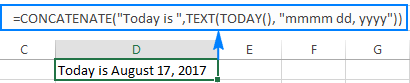### Shortcuts to get today's date in Excel

The inset today's date as an unchangeable timestamp that won't automatically update the next day, use one of the following keyboard shortcuts:

• To inset today's date: Ctrl + ;
• To insert the current time: Ctrl + Shift + ;
• To enter the current date and time: Ctrl + ; then Space and then Ctrl + Shift + ;

## How to use TODAY function in Excel - formula examples

As you already know, the main purpose of the Excel TODAY function is to get today's date. Aside from that, you can use TODAY() in combination with other functions to perform more complex calculations based on the current date. Below you will find a few examples of such formulas.

### Add or subtract days to/from today's date

To add or subtract a specific number of days to/from the current date, use a simple arithmetic operation of addition or subtraction, respectively.

For example, to add 7 days to todays' date, use this formula:

=TODAY()+7

To subtract 7 days from the current date, go with this one:

=TODAY()-7

To exclude weekends (Saturday and Sunday) from your calculations, nest the TODAY function within WORKDAY that deals with weekdays only:

To add 7 workdays to today's date:

=WORKDAY(TODAY(), 7)

To subtract 7 workdays from today's date:

=WORKDAY(TODAY(), -7)

The following screenshot shows the results: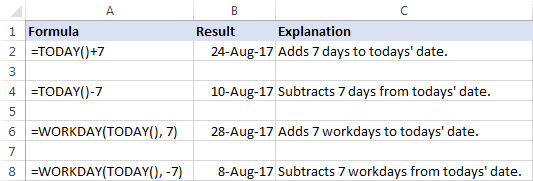Tip. To display the calculated date correctly, set the formula cell's format to Date.

### Get the number of days before or after a certain date

To calculate how many days are left before some data, subtract today's date from the future date you are counting toward:

date-TODAY()

The date can be supplied directly to a formula in the format that Excel can understand, or by using the DATE function, or as a reference to the cell containing the date.

For example, to find out how many days are left till December 31, 2017, use one of these formulas:

`=A2-TODAY()`

`=DATE(2017,12,31)-TODAY()`

`="12/31/2017"-TODAY()`

All three formulas tell us that at the moment of writing (August 17, 2017), 136 days were left till the end of the year 2017: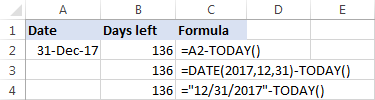To calculate the number of days since a certain date, subtract the past date from today's date:

TODAY()-date

For example, to find out how many days have passed since January 1, 2017, use one of these formulas:

`=TODAY()-A2`

`=TODAY()-DATE(2017,1,1)`

`=TODAY()-"1/1/2017"`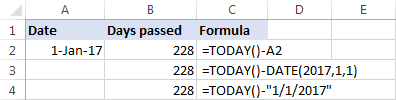Tip. If the result is not displayed correct, be sure to apply the General format to the formula cell.

### Calculate months since or before a certain date

To get the number of months between today and a past date, use the DATEDIF(start_date, end_date, unit) function with the past date in the start_date argument, TODAY() as end_date and "m" unit denoting months:

DATEDIF(past_ date, TODAY(),"m")

To get the number of months between today and a future date, swap the start_date and end_date arguments:

DATEDIF(TODAY(), future-date,"m")

With the date of interest in cell A4, use the following formulas to calculate time difference in the number of complete months:

To calculate months since a certain date in the past:

`=DATEDIF(A4,TODAY(),"m")`

To calculate months before a certain date in the future:

`=DATEDIF(TODAY(),A4,"m")`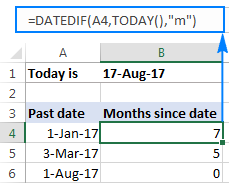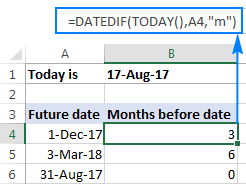### Calculate years since / before a certain date

The formulas to calculate years based on today's date are similar to the ones discussed in the above example. The difference is that you use "y" unit to get the number of complete years between today and another data:

To calculate years since a past date:

DATEDIF(past_ date, TODAY(),"y")

To calculate years before a future date:

DATEDIF(TODAY(), future-date,"y")

Assuming the past/future date is in cell A4, you should be good with using these formulas:

The number of complete years since date:

`=DATEDIF(A4,TODAY(),"y")`

The number of complete years before date:

`=DATEDIF(TODAY(),A4,"y")`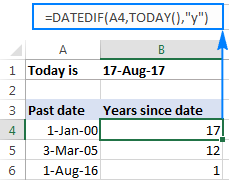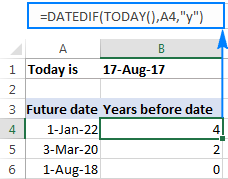### Get age from birthdate

If you know someone's year of birth, you can subtract that year from the current year to find the person's age:

YEAR( TODAY())-year_of_birth

For example, if the person was born in 2000, you use the following formula to get his/her age:

`=YEAR( TODAY())-2000`

Or, you can enter the year of birth in a separate cell and reference that cell in your formula: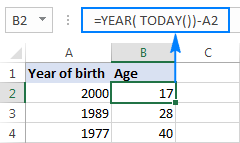You can learn a few other age calculation formulas in this tutorial: How to get age from date of birth in Excel.

### Find the nearest date to today

If you are curious to know which date in a list is closest to today's date, use one of the following array formulas to find it out.

#### Get a past date closest to today

To find a past date nearest to the current date, first "filter out" the dates greater than today, and then use the MAX function to get the largest date among the remaining ones:

MAX(IF(range < TODAY(), range))

With the dates in cells A2 to A10, the formula would go as follows:

`=MAX(IF(\$A\$2:\$A\$10 < TODAY(), \$A\$2:\$A\$10))`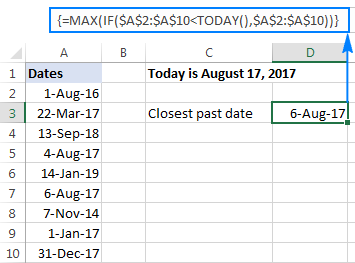#### Get a future date closest to today

To find the nearest future date, identify the dates that are greater than today, and then use the MIN function to get the smallest date among them:

MIN(IF(range >TODAY(), range))

For our sample data set, we'd use this formula:

`=MIN(IF(\$A\$2:\$A\$10 > TODAY(), \$A\$2:\$A\$10))`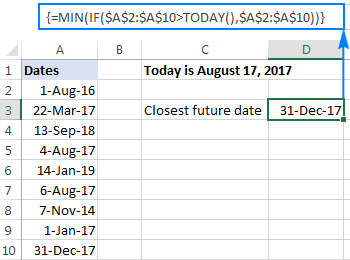#### Get any date closest to today

To get the nearest date in a mixed list of past and future dates, use the classic INDEX MATCH formula with a couple of modifications:

INDEX(range, MATCH(MIN(ABS(range - TODAY())), ABS(range - TODAY()), 0))

Here's how this generic formula works:

• MIN(ABS(range -TODAY())) part. First, you subtract today's date from each date in the range of dates. Then, the ABS function returns the differences as absolute values without regard to their sign. The MIN finds the minimal value, which goes to INDEX MATCH as the lookup value.
• ABS(range -TODAY()) part. You subtract today's date from the range of dates and return an array of absolute values. This array is where INDEX MATCH searches for the lookup value.

In this example, the formula takes the following shape:

`=INDEX(\$A\$2:\$A\$10, MATCH(MIN(ABS(\$A\$2:\$A\$10 - TODAY())), ABS(\$A\$2:\$A\$10 - TODAY()), 0))`

The screenshot below shows the result: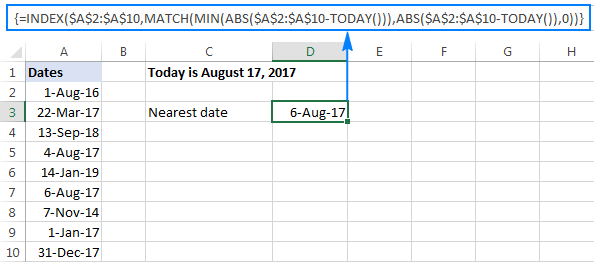Note. All three formulas to get the nearest date are array formulas, so they should be completed by pressing Ctrl + Shift + Enter.

### How to highlight today's date in Excel

When working with a long list of dates or designing your own calendar in Excel, you may want to have the current date highlighted. To have it done, create a conditional formatting rule with this formula:

`=B2=TODAY()`

Where B2 is the left-top-most cell of the range to which the rule applies.

The result may look something similar to this: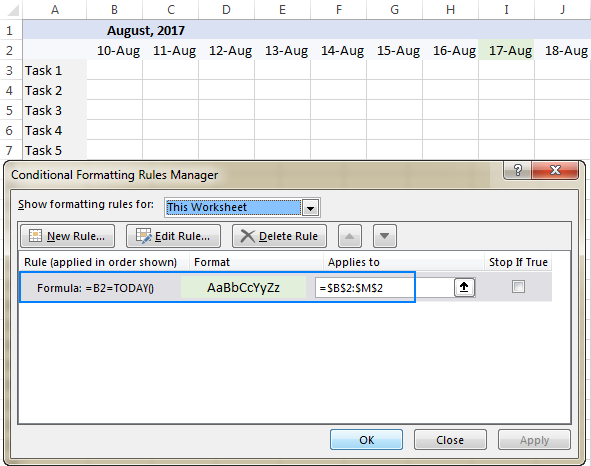The detailed steps to set up a conditional formatting rule can be found here: How to create a conditional formatting rule based on formula.

To have a closer look at the formulas discussed in this tutorial, you are welcome to download our sample workbook below. Thank you for reading!